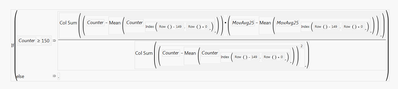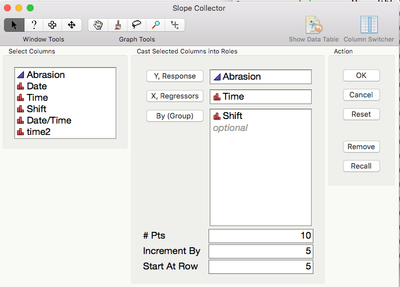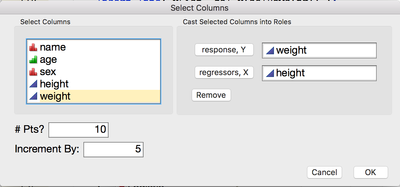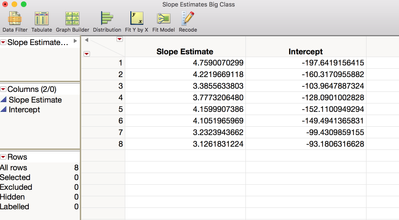It’s World Statistics Day! To honor the theme of the day, the JMP User Community is having conversations about the importance of trust in statistics and data. And we want to hear from you! Tell us the steps you take to ensure that your data is trustworthy.
Choose Language Hide Translation Bar
Highlighted

## Moving Window Slopes

Trying to compute slopes in a moving window.

Y = MovAvg25

X = Counter

Objective is the computation of least square slopes for any row in the data for a moving (sliding) window size of 150 pts.

I believe  function of Index(Row()-149, Row()+0) is correctly describing the moving window (this is before I realized that JMP13 has a moving window function built in, I can use that too) ....

Overall data contains 1800 pts. If I change the moving window size to coincide w/ 1800 (ie. Row()-1799 instead of Row()-149), then the formula computes overall single slope correctly. When using 150 pts, a wrong output gets calculated as a single identical slope for all pts (ie.scalar, hence I know something is wrong).

thanks in advance for any help ....

What I haveor in other form

If( :Counter >= 150,

Col Sum(

(:Counter

-Mean(

:Counter[

Index(

Row() - 149,

Row() + 0

)]

)) * (:MovAvg25

-Mean(

:MovAvg25[

Index(

Row() - 149,

Row() + 0

)]

))

) /

Col Sum(

(:Counter

-Mean(

:Counter[

Index(

Row() - 149,

Row() + 0

)]

)) ^ 2

),

.

)

1 ACCEPTED SOLUTION

Accepted Solutions
Highlightedstan_koprowskiCommunity Manager

## Re: Moving Window Slopes

Hello,

I posted an example of JMP addin that could be used to calculate the moving slope and intercepts for a given x and y with or without a by-variable.  Optionally, you can set the # of points to use in the calculations, how many rows to increment by, and the starting row.Feel free to modify and use for your particular situation.

Thanks for using JMP!

6 REPLIES 6
Highlightedstan_koprowskiCommunity Manager

## Re: Moving Window Slopes

Hi,

Let me see if I understand your question--

You would like to calculate the slope for a fixed number of rows (pts) and repeat using an increment or offset?

Using Bigclass.jmp in the example below:Produces the following table of output:If so, I have a JMP addin that will do what you want.

If not, can you provide a sample data set with the formula and we can help you further.

Thanks,

Stan

Highlighted

## Re: Moving Window Slopes

Stan,

Your addin gets close. I am trying to do what you described except I have many assets that I need to calculate slope indepedently.

Also - I had some counter variable by which I am trying to control when to start calculating the slope.

So, this turns into slope calculation in a window size of 150 moving the windows by 1 point (window size will still need remain constant at 150 for all calculations) ... executed on a per ASSET basis.

Is there a way to do this via a formulae (like I was trying ) .. ?

As an option , I could use your addin, and rejoin data into my main table .However, it must do per Asset. I have over 1000 assets (so manually it becomes difficult)

thanks.stan_koprowskiCommunity Manager

## Re: Moving Window Slopes

Hi,

Let me modify the addin to allow for a by or grouping variable that will allow for the slope to be calculated by asset.

For the counter variable--is this a particular row or observation in the data table that you need to start the calculations from, i.e., instead of all rows beginning with row 1 you would like to specify the starting row?

If so, I can probably add a start from row (counter) variable as well.

Thanks,

Stan

Highlighted

## Re: Moving Window Slopes

Stan,

Sure that would work. By "variable" (i.e. asset) would solve the most important need.

Counter variable main need is to control the slope w/o too much data re-formatting... I need the slope to start executing when there is enough data pts in the beginning. Your "start from" is the right terminology for this. It would have to use the unique value (or first occurence) of a parameter (in my case called "counter"). In my simple case, I am simply incrementing data points by "1" as they are received chronologically in time. So counter data is unique and is always incrementing,

I take it that there is no easy way to describe a moving window slope via a formulae . I think the slope calculation and other related outputs of least squares need to become a built-in function. In fact, I also like to see a Median slope calculation (Siegel slope) as well.

Appreciate and thanks for your support.

Highlightedstan_koprowskiCommunity Manager

## Re: Moving Window Slopes

Hello,

I posted an example of JMP addin that could be used to calculate the moving slope and intercepts for a given x and y with or without a by-variable.  Optionally, you can set the # of points to use in the calculations, how many rows to increment by, and the starting row.Feel free to modify and use for your particular situation.

Thanks for using JMP!

Highlighted

## Re: Moving Window Slopes

Thanks Stanley. I was out of country for a while, sorry it took me some time to get back to this post.

Article Labels

There are no labels assigned to this post.